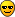# TMultiGraph SetRangeUser

Hello,

I have problems when trying to set the X axis range with a TMultiGraph built of TGraphErrors…
The X axis is in log scale…

Here is a small example reproducing my problem:

``````void TEST()
{
gROOT->Reset();
gStyle->SetCanvasColor(0);
gStyle->SetPalette(1);
gStyle->SetBarOffset(0);

int     N     = 10;
double* S_Eff1 = new double[N];
double* B_Eff1 = new double[N];
double* S_Err1 = new double[N];
double* B_Err1 = new double[N];

double* S_Eff2 = new double[N];
double* B_Eff2 = new double[N];
double* S_Err2 = new double[N];
double* B_Err2 = new double[N];

for(int i=0;i<N;i++){
S_Eff1[i] = 3*pow(1.5,-1*i);
B_Eff1[i] = 1*pow(10,-1*i);
S_Err1[i] = 0.01 * pow(10,-1*i);
B_Err1[i] = 0.01 * pow(10,-1*i);

S_Eff2[i] = 3*pow(1.2,-1*i);
B_Eff2[i] = 1*pow(3,-1*i);
S_Err2[i] = 0.01 * pow(3,-1*i);
B_Err2[i] = 0.01 * pow(3,-1*i);
}

TGraphErrors* Discrim_Same = new TGraphErrors(N, B_Eff1, S_Eff1, B_Err1, S_Err1);
Discrim_Same->SetMarkerStyle(22);
Discrim_Same->SetMarkerColor(8);
Discrim_Same->SetMarkerSize(2);

TGraphErrors* Estim_Same = new TGraphErrors(N, B_Eff2, S_Eff2, B_Err2, S_Err2);
Estim_Same->SetMarkerStyle(22);
Estim_Same->SetMarkerColor(4);
Estim_Same->SetMarkerSize(2);

TCanvas *c1 = new TCanvas("c1","c1",800,600);

TMultiGraph* mg1 = new TMultiGraph();

mg1->Draw("A");
mg1->GetXaxis()->SetRangeUser(1E-15,1);
c1->Update();
}``````

I am trying to fix the range axis to 1E-15 to 1…
I tried to do it on the TMultiGraph or directly on the TGraphErrors but in both cases, nothing happen…

Thanks for help,
Loic

``````{
int     N     = 10;
double* S_Eff1 = new double[N];
double* B_Eff1 = new double[N];
double* S_Err1 = new double[N];
double* B_Err1 = new double[N];

double* S_Eff2 = new double[N];
double* B_Eff2 = new double[N];
double* S_Err2 = new double[N];
double* B_Err2 = new double[N];

for(int i=0;i<N;i++){
S_Eff1[i] = 3*pow(1.5,-1*i);
B_Eff1[i] = 1*pow(10,-1*i);
S_Err1[i] = 0.01 * pow(10,-1*i);
B_Err1[i] = 0.01 * pow(10,-1*i);

S_Eff2[i] = 3*pow(1.2,-1*i);
B_Eff2[i] = 1*pow(3,-1*i);
S_Err2[i] = 0.01 * pow(3,-1*i);
B_Err2[i] = 0.01 * pow(3,-1*i);
}

TGraphErrors* Discrim_Same = new TGraphErrors(N, B_Eff1, S_Eff1, B_Err1, S_Err1);
Discrim_Same->SetMarkerStyle(22);
Discrim_Same->SetMarkerColor(8);
Discrim_Same->SetMarkerSize(2);
Discrim_Same->Draw("ALP");

TGraphErrors* Estim_Same = new TGraphErrors(N, B_Eff2, S_Eff2, B_Err2, S_Err2);
Estim_Same->SetMarkerStyle(22);
Estim_Same->SetMarkerColor(4);
Estim_Same->SetMarkerSize(2);

TCanvas *c1 = new TCanvas("c1","c1",800,600);

TMultiGraph* mg1 = new TMultiGraph();

c1->DrawFrame(1E-15,0.001,1.,3.8);
mg1->Draw();
}``````

it is working, thanks…

But “c1->DrawFrame(1E-15,0.001,1.,3.;”
fix also the Y scale, that I’d like to keep free…

Is there anyway to reduce the Y range to the interested region later?
or to set the X range without adding constraints on the Y one?

thanks again,
Loic

You can leave the Y scale “free” if you took the Y values from your data set and to not impose them like the X ones. That is fairly easy to do (that’s why I did not do it). DrawFrame set both X and Y ranges…